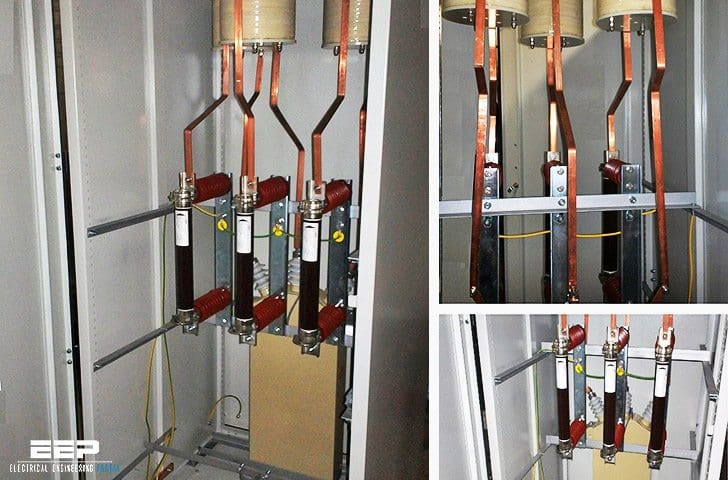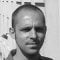Get access to premium HV/MV/LV technical articles, electrical engineering guides and papers. Check our new membership plans and prices!

# Protection of capacitor banks by fuses during energization and operation

Home / Technical Articles / Protection of capacitor banks by fuses during energization and operation

## Stress during bank energization & operation

Stress specific to the protection of capacitor banks by fuses, which is addressed in IEC 60549, can be divided into two types: Stress during bank energization (the inrush current, which is very high, can cause the fuses to age or blow) and Stress during operation (the presence of harmonics may lead to excessive temperature rises).Protection of capacitor banks by fuses (on photo: Fixed 10kV 250kVAr capacitor bank protected by fuses)

The types of stress also vary depending on the type of configuration: single bank or multiple step bank.

Contents:

## 1. Temperature rise

If capacitors are used, because of the harmonics, which cause additional temperature rise, a common rule for all equipment is to derate the rated current by a factor of 30 to 40 %.

This rule applies equally to fuses, which, when combined with the derating required to take into account their installation, results in a coefficient of 1.7 to be applied to the capacitive current in order to determine the appropriate fuse link rating.

Go back to contents ↑

## 2. Inrush current peak

### 2.1 Single capacitor bank

This type of circuit can be illustrated as shown in the diagram in Figure 1, in which:

• L = generator inductance
• R1 = fuse resistance (see appendix 1)
• R2 = resistance of the upstream circuit, calculated based on UN, Isc and cos φ.

When starter D closes, the transient current IT of the load C is applied, where R = R1 + R2, according to the equation:V is the voltage in A on closing. The orders of magnitude of L, R and C mean that the following terms can be ignored: R2 / 4L2 on the basis of which:This transient current is superimposed onto the 50 Hz wave, which results in the current wave form illustrated in figure 2.

The current is at maximum value when V reaches the voltage peak, i.e.:As with transformers, the fuse selection can be validated by checking the relationships between the peak current and the rated current IT / IN as well as the rise time constant. Therefore, if:the IT / IN ratio can be expressed as:andExperience has shown that it is absolutely vital that the fuse does not blow for a current IT during time tT.

Go back to contents ↑

#### Practical example for a single capacitor bank

• UN = 10 kV
• IN bank = 35 A
• Isc = 40 kA (cos φ = 0.1)
• C = 19.3 × 10-6 F

By means of the calculation:

• L = 0.46 × 10-3 H
• R2 = 14.5 × 10-3 Ω.
The rating calculated on the basis of the thermal criteria is: 35 × 1.7 = 60 A. This results in the selection of the following standard rating: IN fuse = 63 A.

In appendix 1:

• R1 = 13 × 10-3 Ω for a fuse rated at 63A / 12kV
• or R = 27.5 × 10-3 Ω

on the basis of which IT = 1670 A and τ = 33.5 × 10-3 s.

A comparison with the time/current characteristics (appendix 2, point A) shows that a rating of 125 A must be selected. Therefore, R1 = 5 × 10-3 Ω and R = 19.5 × 10-3 Ω, on the basis of which IT = 1670 A and τ = 47.2 x 10-3 s.

When recorded on the curves for point (B), this point confirms that a rating of 125 A is indeed correct.

Go back to contents ↑

### 2.2 Multiple step capacitor bank

When the bank in position n is switched on, supposing that the (n-1) other banks have already been switched on, the oscillatory load will be identical. However, in this case, the other banks connected in parallel will act as additional sources of very low internal impedance.

This internal impedance (inductance Li in figure 3) comprises stray inductances from busbars and cables (order of magnitude 0.4 μH/meter) and may also comprise an interference suppressor choke designed to limit the inrush current.

In effect, this current not only poses a danger to the fuse elements, it also compromises the electrical endurance of the switchgear and may even compromise the service life of the capacitors themselves.

As the inrush current flowing via L may be negligible, the inrush current is calculated by applying the following formula:WhereAlthough appearing to be similar to the calculation for the single bank, this calculation is in fact slightly more complicated. You must:

1. Select a fuse link according to the thermal criteria:
2. Calculate:3. Calculate:4. Record the point (IT, τT ) on the time/current characteristics,
5. If necessary, select a different rating, calculate a new RE value and start the verification process again.
If the steps are identical, a single calculation will suffice. However, if they are not, you must investigate several scenarios according to the operating mode of the bank.

Go back to contents ↑

#### Practical example for a multiple step capacitor bank

• 3 banks:
• UN = 10 kV
• IN bank = 35 A
• Isc = 40 kA (cos φ = 0.1)
• Cables = 5 rn or Li = 2 μH

When the third step closes:

• Ci = 19.3 × 10-6 F and L= 1 μH
• Ri = 5 × 10-3 Ω and Rs = 2.5 x 10-3 Ω

On the basis of which:

• CE = 12.9 μF
• LE = 3 × 10-6 H
• RE = 7.5 10-3 Ω
• IT = 16900 A
• τ = 0.8 × 10-3 s

The time/current characteristics do not provide specific data for pre-arc durations of less than a millisecond. A value of I2t constant can be estimated in this zone. If the minimum value of the fuse assembly is assumed to be 125 A (defined in the previous example), this results in the following values:

 IN fuse I2t fusing 125 A 64 × 103 A2 s 160 A 76 × 103 A2 s 200 A 140 × 103 A2 s

The stress applied to the fuse assembly rated at 125 A is:

0.8 × 10-3 × (16900)2 = 228 × 103 A2 s

and even 200 A is unsuitable!

This type of bank cannot be protected in this way using for example Fusarc-CF fuses. In some impossible cases, there is a solution that consists of protecting all three banks with a single common fuse (see figure 4).Figure 4 – Diagram of a bank with three capacitor steps protected by a single fuse

With this type of diagram, two cases should be considered:

Case #1

The three banks cannot be powered up simultaneously. In this case, each time a bank is powered up, the fuse interprets this as the activation of a single bank. A fuse with a rating of 125 A can withstand the inrush current (see the example above).

The main type of stress is thermal stress, which imposes a rated current of 3 × 35× 1.7 = 179A or IN fuse = 200A.

Case #2

All three banks can be powered up simultaneously. The system becomes equivalent to a single bank with triple power, i.e.:

• L = 0.46 × 10-3 H
• C = 57.9 × 10-6 F
• IT = 2900A
Assuming a fuse with a rating of 200 A, (R1 = 2.5 × 10-3 Ω) where R2 = 14.5 × 10-3 Ω (see above) τ = 54 × 10-3 s

Point C, appendix 2, shows that 200 A is suitable in the majority of cases. Paradoxically, in this case, it is possible to protect all three banks globally using one fuse despite the fact that protecting each individually is impossible.

Go back to contents ↑

### Appendix 1: Cold resistance of Fusarc CF fuses

Go back to contents ↑

### Appendix 2: Time/current characteristics in the Fusarc CF range of fuses (Schneider Electric)

Go back to contents ↑

Reference // Design and use of MV current-limiting fuses by O. Bouilliez and J.C. Perez Quesada

Get access to premium HV/MV/LV technical articles, electrical engineering guides, research studies and much more! It helps you to shape up your technical skills in your everyday life as an electrical engineer.### Edvard Csanyi

Electrical engineer, programmer and founder of EEP. Highly specialized for design of LV/MV switchgears and LV high power busbar trunking (<6300A) in power substations, commercial buildings and industry facilities. Professional in AutoCAD programming.

1.Sar Samnang
May 24, 2017

Dear Sir,
I need you to solve my problem that i don’t have reference document to do:
01-Load estimate of Lighting power densities and power socket densities(per W/m2) using the building area method.
02-The selection of illuminance levels in work place or other area. Could you help me?

Thanks

2.Olivier Bouilliez
May 01, 2017

Hi Edvard… in case of multiple banks still protected by fuses, a smart solution is to replace the nice copper bars we see on the picture by… steel.. on a meter or so. The heat dissipation is minimal, but the limitation effect on inrush current is surprising…ans allows to still use fuses without them to age.
Seems counter intuitive, but it works.

•Edvard
May 06, 2017

Thank you for your comment Olivier. Is this a practice, to use steel bars instead of copper? I haven’t seen this…

### Subscribe to Weekly Digest

Get email alert whenever we publish new electrical guides and articles.